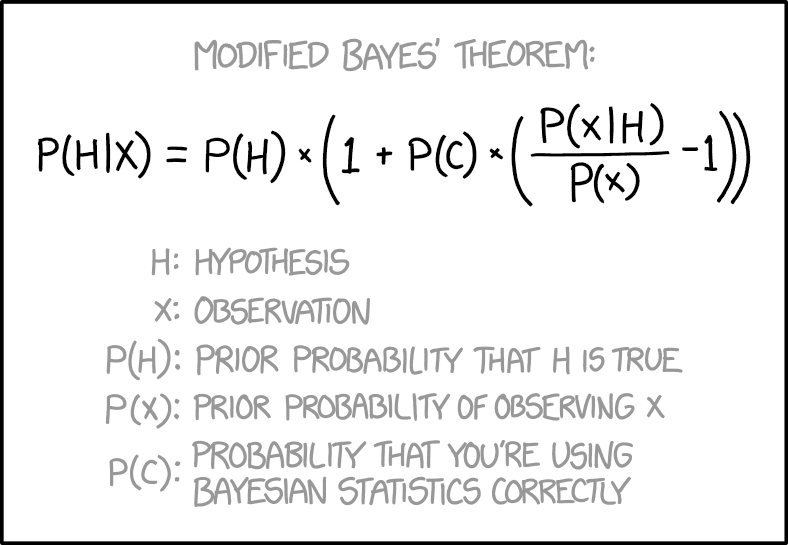# 贝叶斯定理 #

## 形式一：两个事件之间的关联 #

\begin{aligned}\Pr(A \mid B)\times\Pr(B) &= \Pr(B \mid A)\times\Pr(A)\\ \Pr(A \mid B) &= \frac{\Pr(B \mid A)\times\Pr(A) }{\Pr(B)}\\ &= \frac{\Pr(B \mid A)\times\Pr(A) }{\Pr(B \mid A)\times\Pr(A) + \Pr(B \mid \lnot A)\times\Pr(\lnot A)}\end{aligned}

• A 的先验概率：$\Pr(A)$
• A 的后验概率：$\Pr(A \mid B)$
• B 的先验概率：$\Pr(B)$
• B 的后验概率：$\Pr(B \mid A)$

### 事件 B 发生的总概率 #

\begin{aligned}\Pr(B) &= \phantom{111} \Pr(A\cap B)\phantom{(11)} + \phantom{111}\Pr(\lnot A\cap B)\\ &= \overbrace{\Pr(B \mid A)\times\Pr(A)} + \overbrace{\Pr(B \mid \lnot A)\times\Pr(\lnot A)} \end{aligned}

• A 的先验概率：$\Pr(A)$$\Pr(\lnot A)=1-\Pr(A)$
• 如果 A 发生，那么 B 发生的概率：$\Pr(B \mid A)$
• 如果 A 不发生，那么 B 发生的概率：$\Pr(B \mid \lnot A)$

### 事件 B 发生了，那么事件 A 发生的概率？ #

\begin{aligned}\Pr(A \mid B) &= \frac{\Pr(B \mid A)\times\Pr(A) }{\Pr(B)}\\ &= \frac{\Pr(B \mid A)\times\Pr(A) }{\Pr(B \mid A)\times\Pr(A) + \Pr(B \mid \lnot A)\times\Pr(\lnot A)} \\ &= \frac{\Pr(A\cap B)}{\Pr(A\cap B) + \Pr(\lnot A\cap B)}\end{aligned}

~ 智识分子. 万维钢. Part III. 贝叶斯定理的胆识.

“Extraordinary claims require extraordinary evidence” (ECREE)

## 形式二：通过信号区分多种情况（贝叶斯更新） #

$$\Pr(\theta_A \mid s) = \frac{\Pr(\theta_A)\times \Pr(s \mid \theta_A)}{\Pr(\theta_A)\times \Pr(s \mid \theta_A) + \Pr(\theta_B)\times \Pr(s \mid \theta_B)}$$

• 可能出现的情况（例如是否生病）：$\theta_A$（生病）， $\theta_B$（健康）
• 观察到的信号（例如检测）：$s$（检测为阳性）
• 分母为 $s$ 的全概率公式

• 情况的先验：$\Pr(\theta_A)$（得病率），$\Pr(\theta_B)$
• 信号的准确度：$\Pr(s \mid \theta_A)$（灵敏度），$\Pr(s \mid \theta_B)$（1 - 特异度）

### 同样信号时不同情况的比值比，及线性形式（log odds form） #

\begin{aligned}\frac{\Pr(\theta_A \mid s)}{\Pr(\theta_B \mid s)} &= \frac{\frac{\Pr(\theta_A)\times \Pr(s \mid \theta_A)}{\Pr(\theta_A)\times \Pr(s \mid \theta_A) + \Pr(\theta_B)\times \Pr(s \mid \theta_B)}}{\frac{\Pr(\theta_B)\times \Pr(s \mid \theta_B)}{\Pr(\theta_A)\times \Pr(s \mid \theta_A) + \Pr(\theta_B)\times \Pr(s \mid \theta_B)}} \\ &= \frac{\Pr(\theta_A)\times \Pr(s \mid \theta_A)}{\Pr(\theta_B)\times \Pr(s \mid \theta_B)}\end{aligned}

$$\log\left(\frac{\Pr(\theta_A \mid s)}{\Pr(\theta_B \mid s)}\right) = \log\left(\frac{\Pr(\theta_A)}{\Pr(\theta_B)}\right) + \log\left(\frac{\Pr(s \mid \theta_A)}{\Pr(s \mid \theta_B)}\right)$$

## 娱乐 #